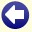## Solution to: Long Division

We will indicate the numbers in the long division in the manner shown below:

```       . . . / . . . . . . . . \ . . 8 . .             A /  B      \ C
. . .                                      D
-------                                     ---
. . . .                                    E
. . .                                    F
-------                                   ---
. . . .                                  G
. . . .                                  H
-------                                 ---
0                                  0
```

For E and G two digits have been brought down, therefore both the second and the fourth digits of C are 0.

Because F consists of three digits and equals the outcome of 8 times A, A must be smaller than 1000/8=125. Number H consists of four digits and is the product of number A and the last digit of C. Therefore, H is at least 1000, and because A is smaller than 125, the last digit of C must be 9. Moreover, A must be at least 1000/9, so be larger than 111.

Because the number D is subtracted from a number of four digits and the outcome consists of two digits, the first digit of D must be 9. This is possible only if the first digit of C is 8.

Now we know that the number C equals 80809 and number B is at least 10000000. From this, it follows that A is at least 124. Because we also know that A lies between 111 and 125, A equals 124.

The complete long division can now be filled in:

```       1 2 4 / 1 0 0 2 0 3 1 6 \ 8 0 8 0 9
9 9 2
-------
1 0 0 3
9 9 2
-------
1 1 1 6
1 1 1 6
-------
0
```Back to the puzzle
This website uses cookies. By further use of this website, or by clicking on 'Continue', you give permission for the use of cookies. If you want more information, look at our cookie policy.